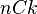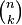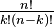# Combination (mathematics) facts for kids

Kids Encyclopedia Facts

In mathematics, combination is used for picking a number of objects from a given set of objects. Combinatorics looks at the number of possibilities to pick k objects from a set of n. It does not take into account the order in which these are picked. People talk about finding permutations if the order in which the objects are picked matters. This explains why there are more permutations than combinations. One combination, picking the objects 1,3, and 5 in the example can be done in the following ways: {1,3,5}, {1,5,3}, {3,1,5}, {3,5,1}, {5,1,3} and {5,3,1}.

In general, the number of combinations of k objects from n objects, written as$nCk$ or$\tbinom{n}{k}$, is equal to$\tfrac{n!}{k!(n-k)!}$, where "!" stands for the factorial notation. This number is also known as the binomial coefficient.

## Related pagesCombination (mathematics) Facts for Kids. Kiddle Encyclopedia.